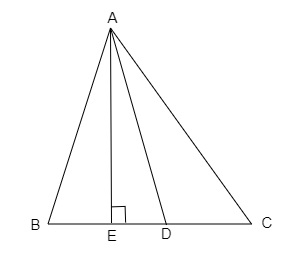"
">

# In figure below, D is the mid-point of side BC and $AE \perp BC$. If $B C=a, A C=b, A B=C, E D=x, A D=p$ and $A E=h,$ prove that $b^{2}=p^{2}+a x+\frac{a^{2}}{4}$."

Given:

In the given figure, D is the mid-point of side BC and $AE \perp BC$.

$B C=a, A C=b, A B=C, E D=x, A D=p$ and $A E=h$.

To do:

We have to prove that $b^{2}=p^{2}+a x+\frac{a^{2}}{4}$.

Solution:

In $\triangle AED$, by using Pythagoras theorem,

$AD^2=AE^2+ED^2$

$AE^2=AD^2-ED^2$.....(i)

In $\triangle AEC$, by using Pythagoras theorem,

$AC^2=AE^2+EC^2$
$b^2=(AD^2-ED^2)+(ED+DC)^2$     (From (i))

$b^2=AD^2-ED^2+ED^2+DC^2+2ED\times DC$

$b^2=AD^2+DC^2+2DC\times ED$

$b^2=p^2+(\frac{a}{2})^2+2\times(\frac{a}{2})\times x$   (Since $DC=\frac{BC}{2}$)
$b^2=p^2+\frac{a^2}{4}+ax$

Hence proved.

Updated on: 10-Oct-2022

45 Views